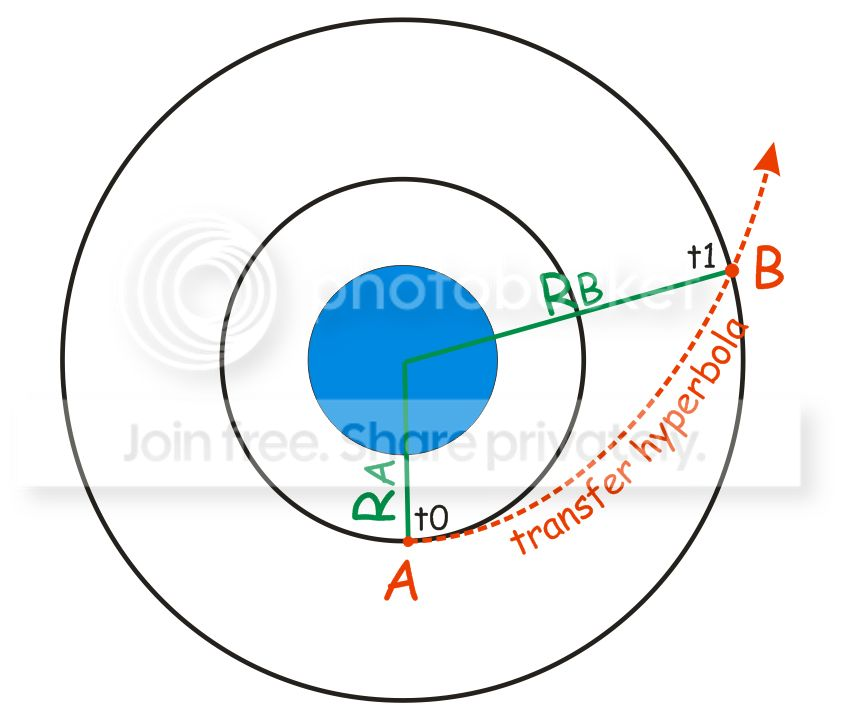# SMa and eccentricity of a hyperbola from TOF and distance

#### dgatsoulis

##### ele2png userIn the pic above we have two spacecrafts in orbits A and B. The orbits are circular and coplanar.

We are given the radii of orbits A and B and the TOF=t1-t0 that it takes to get from A to B via a hyperbolic transfer trajectory.
Are these enough to calculate the semi major axis or the eccentricity of the transfer hyperbola? If yes, how?

I have been reading the equations for hyperbolic orbits but I seem to be going in circles, ending up with equations with two unknowns and not being able to "isolate" one of them. Once I have either of these two unknowns, I can calculate the other one very easily.

#### perseus

But in the absence of other responses I hope this serves you answer something.
But if a method that could be solved by iterations on a computer

Solution with the Ra condition at Perigee

The equation must be solved numerically, using the method of iteration.

υ change from 0 to π (pi)

V1= ((1-cos(υ)).Rb.GM/(Ra2 -Ra.Rb. cos(υ)))

w=GM

V1=SQR((1-cos(υ)).Rb.GM/(Ra2 -Ra.Rb. cos(υ)))

h=V1.Ra

z=V1 h-W

u = true anomaly

T= Time perigee to R(u)

T=h^3.((Z.sin(υ)/((w+z).(z-w).(z.cos(υ))+w)-((w.(2.wtn(2.z.sin(υ)/(2.z.cos(υ)+(sqr(w-z)+sqr(w+z))^2- υ)/((w+z)^(3/2)) .((w-z)^(3/2)))))

If T=(T1-T0) the end else υ= υ+000.1 repeat

After obtaining υ
Rper = (V1.Ra) 2 / (2.GM-V12. Ra)

Parameters of the hyperbola or conical

a = Rper+R1
c = (Rper-R1)/2
Interestingly the problem, maybe someone has other solutions.

#### dgatsoulis

##### ele2png user
Thanks a lot perseus, this is very similar to what my latest approach was.
Since I already know the time, I can narrow in on the cos(u), until I find a solution for T = t1-t0. Then I can find the rest.

This will do just fine, unless perhaps someone else knows a direct analytical solution.

Thanks again.
:tiphat: